# Test: Mathematics-5

## 20 Questions MCQ Test CTET ( Central Teacher Eligibility Test ) Mock Test Series | Test: Mathematics-5

Description
Attempt Test: Mathematics-5 | 20 questions in 20 minutes | Mock test for Teaching preparation | Free important questions MCQ to study CTET ( Central Teacher Eligibility Test ) Mock Test Series for Teaching Exam | Download free PDF with solutions
QUESTION: 1

### A can-do 75% of a job in 18 days and B can do 25% of the job in 12 days. If they work on it together, in how many days can they do 75% of the job?

Solution: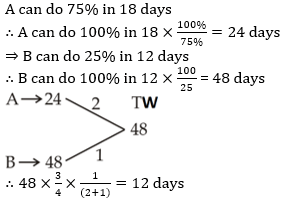QUESTION: 2

### At 30% discount, the selling price of an article is Rs 1050. What is the selling price (in Rs) if the discount is 15%?

Solution: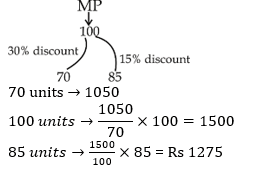QUESTION: 3

### What is the average of all numbers between 9 and 90 which are divisible by 8?

Solution: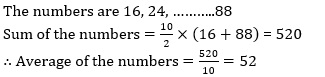QUESTION: 4

A trader had 630 kgs of rice. He sold a part of it at 15% profit and the rest at 8% profit, so that he made a total profit of 12%. How much rice (in kgs) did he sell at 8% profit?

Solution: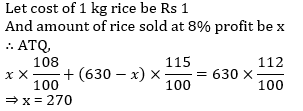QUESTION: 5

Excluding stoppages, the average speed of a train is 120 kmph and including stoppages, it is 50 kmph. For how many minutes does the train stop per hour?

Solution: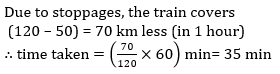QUESTION: 6

The simple and compound interest that can be earned in two years at the same rate is Rs 4000 and Rs 4180 respectively. What is the rate (percent per annum) of interest?

Solution: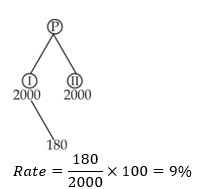QUESTION: 7

6 times of a fraction is greater than 7 times of its reciprocal by 11. What is the fraction?

Solution: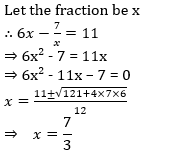QUESTION: 8

A watch having marked price as Rs 8400 is sold for Rs 5040 after two successive discounts. If the first discount is 25% then what is the numerical value of second discount percentage?

Solution: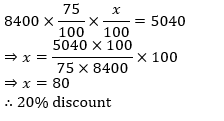QUESTION: 9

Average age of 20 students is 21 years. 2 students leave the group and 1 new student joins the group. The average now becomes 20 years. If age of one of the student who left the group is 26 years and the one who joined is 20 years, then what is the age (in years) of the other student who left the group?

Solution: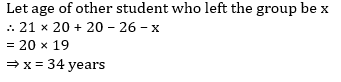QUESTION: 10

A is 1.5 times efficient than B therefore takes 8 days less than B to complete a work. If A and B work on alternate days and A works on first day, then in how many days the work will be completed?

Solution: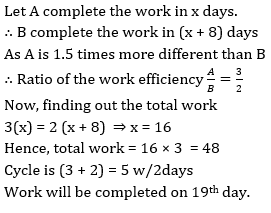QUESTION: 11

A shopkeeper sells a table at a discount of 20% and earns a profit of 60%. If he sells the same table at 40% discount, then what will be his new profit percent?

Solution: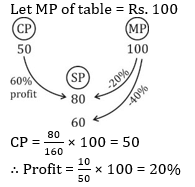QUESTION: 12

5 years ago the average age of a family which includes father, mother and a son was 35 years. 3 years ago the average age of father and mother was 46 years. What is the present age (in years) of the son?

Solution: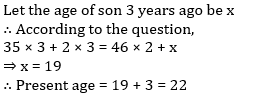QUESTION: 13

The cost price of 60 articles is same as the selling price of x articles. If there is a profit of 20%, then what is the value of x?

Solution: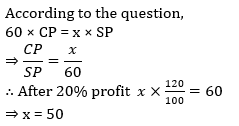QUESTION: 14

A person scores 45% of the total marks in the exam and still fails by 40 marks. The passing percentage of the exam is 55%. What is the maximum marks of the exam?

Solution: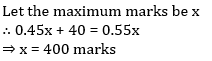QUESTION: 15

A man starts running from point P at 11:00 a.m. with a speed of 10 km/hr. He runs for 2 hours and then takes a 1 hour rest. He continues the pattern till he is caught by another man who starts at 2:00 p.m. from point P and runs non-stop at a speed of 15 km/hr towards the first man. At what time (in p.m.) will the first man be caught?

Solution: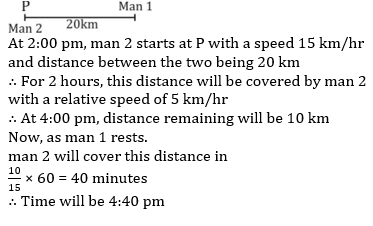QUESTION: 16

The difference of compound interest and simple interest for 3 years and for 2 years are in ratio 23: 7 respectively. What is rate of interest per annum (in %)?

Solution: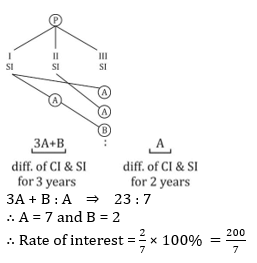QUESTION: 17

A fraction becomes 6/5 when 5 is added to its numerator and becomes 1/2 when 4 is added to its denominator. What will be the value of the fraction?

Solution: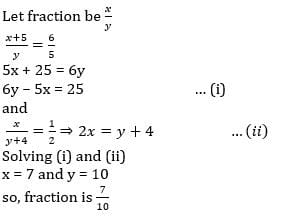QUESTION: 18

Amit can complete a work in 25 days and Punit can complete the same work in 20 days. Punit alone worked at it for 10 days and then left the work. In how many days will Amit alone complete the remaining work?

Solution: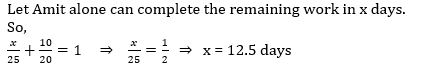QUESTION: 19

The measure of the four successive angles of a quadrilateral are in the ratio 7 : 11 : 7 : 11. The quadrilateral is a ______.

Solution:

Pair of opposite angles are equal in a parallelogram. Rectangle and square are also parallelogram but they have 90° angle at each corner.

QUESTION: 20

What is the discount percentage offered on a book having marked price Rs 2150 being sold at Rs 1892?

Solution: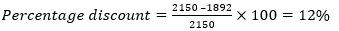Use Code STAYHOME200 and get INR 200 additional OFF Use Coupon Code